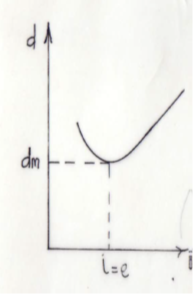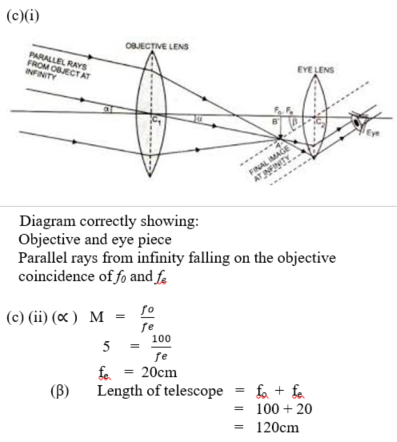Question 10

1. What is a wave front?
2. (i)  State two practical uses of glass prism

(ii) List two factors that determine the deviation of a ray of light travelling from air into a triangular prism.
(iii) Sketch a graph to illustrate the variation of the angle of deviation, d, with that of incidence, i, for a ray of light travelling from air into a triangular glass prism. Indicate on the graph the point at which the angle of incidence i is equal to the angle of emergence e.

1. (i) Draw and label a diagram of an astronomical telescope in normal adjustment.

(ii) The angular magnification of an astronomical telescope in normal adjustment is 5. If the focal length of the objective is 100 cm, calculate the:
(α) focal length of the eyepiece;
(β) length of the telescope.

Observation

Part (a):     This question was popular among candidates but their performance was low. Majority attempted but most candidates proved ignorant about wavefront with incorrect response.

Part (b)(i): Performance was fair as many candidates misinterpreted the question and so wrongly mentioned spectrometer, binocular, periscope.

(ii): Performance was low. The responses showed little or no knowledge of the concept of deviation.
(iii) Performance was low. Several candidates failed to earn full marks from the graph. Some drew a V-shaped graph, others a cylindrical with hemispherical base instead of a curve with a defined minimum point, dm , where angle of incidence, i , equals angle of emergence, e.
Part (c)(i): Most of the candidates were unable to draw the correct diagram while few failed to earned the full marks because fo and fe did not coincide; fo<fe; both optical centres were not co-axial and light rays impinging on lo were not parallel. Performance was low.
(ii)(α) (β): Very few candidates attempted the questions yet with very low outcome

10.    (a) Wave front
A surface over / on which particles vibrate in (the same) phase
(b)(i)  Glass Prisms
Any correct 2 x 1 mark       =
e.g  - Changing direction of light/ dispersion (spectrometer).
-   Viewing distant objects (Binocular)
-   Viewing objects over obstacles (Periscope)
-   Determining refractive index of glass

(ii)     Deviation of a ray in a triangular prism
Any correct 2 x  ½  mark  =
e.g   -   angle of incidence
-   refracting angle of the prism
-   refractive index of the prism
(iii)     GraphAxes correctly distinguished
Correct shape of graph

At dm, i = e correctly shown